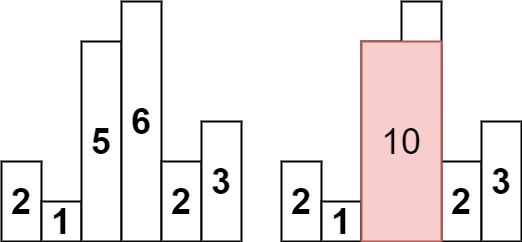572. Largest Rectangle in Histogram

0

Hard

Given an array of integers heights representing the histogram's bar height where the width of each bar is 1, return the area of the largest rectangle in the histogram.Input Format

First Line contains size of input array
Second Line contains Array

Output Format

Print Largest Area

Example

Input

6 2 1 5 6 2 3

Output

10

Constraints

1 <= heights.length <= 105
0 <= heights[i] <= 104